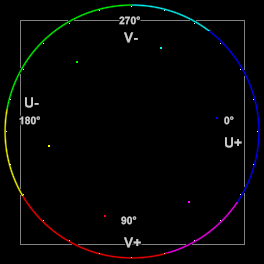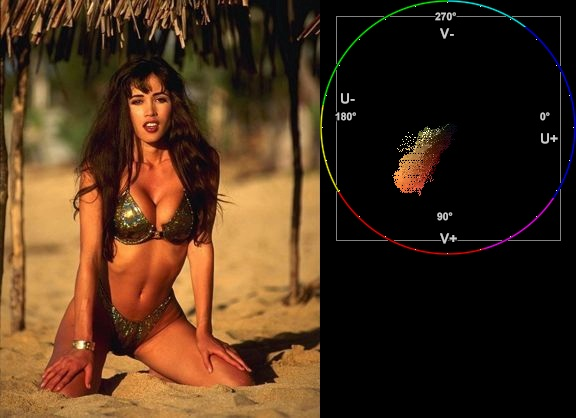# MaskHS

(diff) ← Older revision | Latest revision (diff) | Newer revision → (diff)
Jump to: navigation, search

Returns a mask (as Y8) of clip using a given hue and saturation range. Added in v2.6.

AVS+ adds new realcalc parameter.

## Syntax and Parameters

MaskHS(clip [, int startHue, int endHue, int maxSat, int minSat, bool coring ] )

MaskHS(clip [, int startHue, int endHue, int maxSat, int minSat, bool coring, bool realcalc ] ) AVS+

int  startHue = 0
int  endHue = 360
The resulting mask will contain source values in the range [startHue, endHue] when startHue<endHue. Note that the hue is periodic, thus a hue of 360 degrees corresponds with a hue of zero degrees.
If endHue<startHue then the range [endHue, 360] and [0, startHue] will be selected (thus anti-clockwise). If you need to select a range of [350, 370] for example, you need to specify startHue=370, endHue=350.
Range 0 to 360 (degrees), default 0, 360; thus when using the default values, all pixels will be processed.
The following shows some arbitrary startHue and endHue values for the basic colors, with a Histogram vectorscope to illustrate the color circle:
 Color startHue (center) endHueRed 075 102 115 Yellow 150 176 180 Green 191 230 258 Cyan 279 282 300 Blue 316 000 004 Magenta 028 048 052
int  maxSat = 150
int  minSat = 0
The resulting mask will contain source values in the range [minSat, maxSat].
Practically the saturation of a pixel will be in the range [0,100] (thus 0-100%), since these correspond to valid RGB pixels (100% corresponds to R=255, G=B=0, which has a saturation of 119). An overshoot (up to 150%) is allowed for non-valid RGB pixels (150% corresponds to U=V=255, which has a saturation of sqrt(1272+1272) = 180).
AVS+ TODO needs documentation of behavior with Deep Color formats.
Range 0 to 150 (percent), default 150, 0; thus when using the default values all pixels will be processed.
bool  coring = true
When set to true, the luma (Y) is clipped to TV-range; when set to false, the luma is left untouched.
bool  realcalc = false
AVS+ When true, force 'no-lookup': pure float calculation of new pixel values (always true for certain Deep Color formats) (TODO - clarify)

## Examples

• Suppose we want to create a mask of the skin of the girl below. The proper way to do this is to look at the vectorscope of Histogram:
 ```clip = ... Histogram(clip, mode="color2") ```and estimate the hue range you want to select. As can be seen, the orange hue is between (about) 105 and 165 degrees.
Note: axis labels have been added to the vectorscope as a guide – they are not generated by the above script
Start with a wide hue range and narrow it until the output of MaskHS isolates the range of interest. You can also use Tweak to preview the affected range (with sat=0), as the arguments are compatible.
In our example we end at startHue=105, endHue=138, and the following mask is obtained:
 ```clip = ... MaskHS(startHue=105, endHue=138) ```• Looking at the blue screen example in Overlay the following can be used
```testcard = ColorBars

# example subtitle file with blue background:
subs = ImageSource("F:\TestClips\blue.jpg").ConvertToYV24

# subs.Histogram(mode="color2").ConvertToRGB # blue in [345,359]
mask_hs = subs.MaskHS(startHue=340, endHue=359).Levels(0, 1, 255, 255, 0)

Overlay(testcard, subs, mask=mask_hs, mode="blend", opacity=1)
```

## Changelog

 v2.60 Initial Release